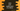# Java program to find Saddle point of a MatrixIn this tutorial, we will learn how to find the saddle point of a matrix in Java. I will give you two examples. Try to run the program with different examples and let me know if you have any query.

### What is Saddle point of a Matrix :

Saddle point is an element of a matrix which is smallest in that row and largest in that column. Let me show you with a example :

``````4 5 2
5 1 1
0 1 0``````

In the above example, for ‘2’, It is the smallest element for the first row. Again it is also the largest element for the third column . So, 2 is a saddle point. A matrix can have more than one saddle point.

### How to find a saddle point in a Matrix ?

Aah..it is little bit complex. Before explaining the whole algorithm, let me tell you that the below algorithm is written by me , so if you think any way to improve it , please please let me know.I have commented the whole code, so maybe it would not be difficult to understand.

### Steps :

1. Pass the ‘matrix’, count of row and count of column to the method findSaddlePoint
2. Scan through each row and find out the smallest element
3. Save the first element of that row as smallest
4. Now iterate through other elements and find for any element less than it i.e. find the smallest element
5. If any equal element is found, scan other elements and if it is the smallest, then check if it is largest or not for this column. If this element is both largest in column and smallest in row, print it as saddle point.
6. After the loop is completed, check for the minimum element if it is largest in the column.
7. For checking if an element is largest or not in a column, we are using checkColumnMax method.
``````/*
*
* you may not use this file except in compliance with the License.
* You may obtain a copy of the License at
*
*
* Unless required by applicable law or agreed to in writing, software
* WITHOUT WARRANTIES OR CONDITIONS OF ANY KIND, either express or implied.
* See the License for the specific language governing permissions and
*/

/**
* Class to find saddle point of a matrix
*/

static void print(String value) {
System.out.println(value);
}

/**
* This method will check maximum value in a specific column and compare it with a given value.
* i.e. it will compare minimum value of a row with all elements of that element's column and check if it is
* maximum or not for that column . If yes, it is a saddle point.
* Return true if it is a saddle point. false otherwise
*
* @param mat               : Given matrix
* @param minValColPosition : coloum position for which we need to check
* @param minValueRow       : minimum value for that Row we have found
* @param rowSize           : total no of row
* @return true or false
*/
static boolean checkColumnMax(int[][] mat, int minValColPosition, int minValueRow, int rowSize) {
//first, set the value as maximum
int maxValCol = minValueRow;

//iterate through each element for that column
for (int i = 0; i < rowSize; i++) { if (mat[i][minValColPosition] > maxValCol) {
//update maximum value if any value is greater than the stored maximum
maxValCol = mat[i][minValColPosition];
}
}
if (maxValCol == minValueRow) {
//if maximum value is same as the value given , return true. i.e. it is a saddle point
return true;
}
return false;
}

/**
* Main method to find saddle point
*
* @param mat : given matrix
*/
static void findSaddlePoint(int[][] mat, int rowSize, int colSize) {

//scan through each row and find out the smallest element for the row
for (int row = 0; row < rowSize; row++) {

int minValueRow = mat[row]; //storing the first element
int minValColPosition = 0;

for (int col = 1; col < colSize; col++) { //iterate through other elements of the row and check for min
// value
if (mat[row][col] < minValueRow) {
minValueRow = mat[row][col];
minValColPosition = col;
} else if (mat[row][col] == minValueRow) { //if minimimum value stored is equal to another element, i
// .e. two values are present. Check for this element if it is a saddle point or not . But first
// confirm this is the minimum value or not .

boolean isMin = true;

// compare with other elements if it is actually a minimum value
for (int i = col + 1; i < colSize; i++) {
if (mat[row][i] < minValueRow) {
isMin = false;
}
}
if (isMin) {
//if it is minimum, check it is maximum for that column or not
if (checkColumnMax(mat, col, minValueRow, rowSize)) {
print("Saddle Point " + "[" + row + ":" + col + "]" + " = " + minValueRow);
}
}
}
}

//check if the minimum value is maxim or not for this column
if (checkColumnMax(mat, minValColPosition, minValueRow, rowSize)) {
print("Saddle Point " + "[" + row + ":" + minValColPosition + "]" + " = " + minValueRow);
}
}

}

public static void main(String[] args) {

print("For the first matrix :");
int mat[][] = {{ 2,  2,  1, 1,  0},
{ 1,  1,  1, 1, -1},
{-1, -1, -1, 0, -2},
{ 0,  0,  0, 0, -4}};

print("For the second matrix :");

int mat1[][] = {{0,  1,  0},
{-1, -2, -3},
{ 0,  1,  0}};

}
}``````

### Output :

``````For the first matrix :